Courses

# Notes | EduRev

## Class 10 : Notes | EduRev

The document Notes | EduRev is a part of the Class 10 Course Class 10 Mathematics by VP Classes.
All you need of Class 10 at this link: Class 10

Page No. 221 - 222
In each of the following, give also the justification of the construction:

Q 1. Draw a circle of radius 6 cm. From a point 10 cm away from its centre, construct the pair of tangents to the circle and measure their lengths.
Sol. Steps of construction:
I. With O as centre and radius 6 cm, draw a circle.
II. Take a point P at 10 cm away from the centre.
III. Join O and P.
IV. Bisect OP at M.
V. Taking M as centre and MP or MO as radius, draw a circle.
VI. Let the new circle intersects the given circle at A and B.
VII. Join PA and PB.
Thus, PA and PB are the required two tangents.
By measurement, we have: PA = PB = 9.6 cm.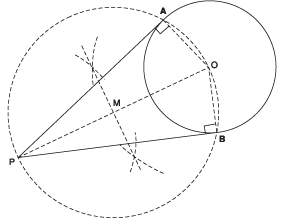Justification:
Join OA and OB
Since PO is a diameter.
∴∠OAP = 90° = ∠OBP    [Angles in a semicircle]
Also, OA and OB are radii of the same circle.
⇒ PA and PB are tangents to the circle.

Q 2. Construct a tangent to a circle of radius 4 cm from a point on the concentric circle of radius 6 cm and measure its length. Also verify the measurement by actual calculation.
Sol. Steps of construction:
I. Join PO and bisect it such that the midpoint of PO is represented by M.
II. Taking M as centre and OM or MP as radius, draw a circle such that this circle intersects the circle (of radius 4 cm) at A and B.
III. Join A and P.
Thus, PA is the required tangent.
By measurement, we have: PA = 4.5 cm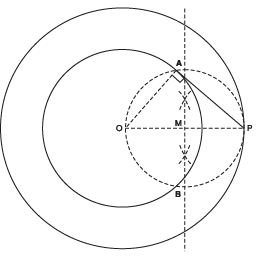Justification:
Join OA such that ∠PAO = 90°  [Angle in a semi-circle]
⇒ PA ⊥ OA
∵ OA is a radius of the inner circle.
∴ PA has to be a tangent to the inner circle.

Q 3. Draw a circle of radius 3 cm. Take two points P and Q on one of its extended diameters each at a distance of 7 cm from its centre. Draw tangents to the circle from these two points P and Q.
Sol. Steps of construction:
I. Join P and O.
II. Bisect PO such that M be its mid-point.
III. Taking M as centre and MO as radius, draw a circle. Let it intersect the given circle at A and B.
IV. Join PA and PB.
Thus, PA and PB are the two required tangents from P.
V. Now, join O and Q.
VI. Bisect OQ such that N is its midpoint.
VII. Taking N as centre and NO as radius, draw a circle. Let it intersect the given circle at C and D.
VIII. Join QC and QD.
Thus, QC and QD are the required tangents to the given circle.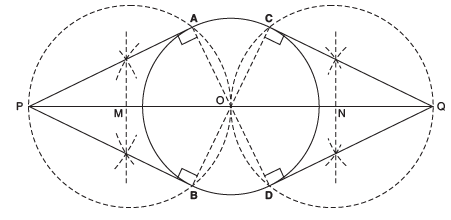Justification:
Join OA such that ∠OAP = 90° [Angle in a semi-circle] ⇒
PA ⊥ OA ⇒ PA is a tangent.
Similarly, PB ⊥ OA ⇒ PB is a tangent
Now, join OC such that ∠QCO = 90° [Angle in a semi-circle]
⇒ QC ⊥ OC
⇒ QC is a tangent.
Similarly, QD ⊥ OC ⇒ QD is a tangent.

Q 4. Draw a pair of tangents to a circle of radius 5 cm which is inclined to each other at an angle of 60°.
Sol. Steps of construction:
I. With centre O and radius = 5 cm, draw a circle.
II. Draw an angle ∠AOB = 120°.
III. Draw a perpendicular on OA at A.
IV. Draw another perpendicular on OB at B.
V. Let the two perpendiculars meet at C.
CA and CB are the two required tangents to the given circle which are inclined to each other at 60°.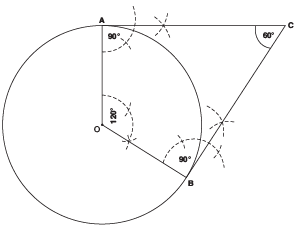Justification:
In a quadrilateral OACB, using angle sum property, we have:
120° + 90° + 90° + ∠ACB = 360°
⇒ 300° + ∠ACB = 360°
⇒ ∠ACB = 360° - 300° = 60°.

Q 5. Draw a line segment AB of length 8 cm. Taking A as centre, draw a circle of radius 4 cm and taking B as centre, draw another circle of radius 3 cm. Construct tangents to each circle from the centre of the other circle.
Sol. Steps of construction:
I. Bisect the line segment AB. Let its mid point be M.
II. With centre as M and MA (or MB) as radius, draw a circle such that it intersects the circle with centre A at the points P and Q.
III. Join BP and BQ.
Thus, BP and BQ are the required two tangents from B to the circle with centre A.
IV. Let the circle with centre M, intersects the circle with centre B at R and S.
V. Join RA and SA.
Thus, RA and SA are the required two tangents from A to the circle with centre B.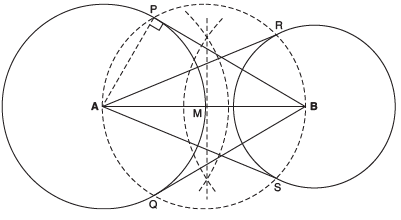Justification:
Let us join A and P.
∵ ∠APB = 90°    [Angle in a semi circle]
∴ BP ⊥ AP
But AP is radius of the circle with centre A.
⇒ BP has to be a tangent to the circle with centre A.
Similarly, BQ has to be tangent to the circle with centre A.
Also, AR and AS have to be tangent to the circle with centre B.

Q 6. Let ABC be a right triangle in which AB = 6 cm, BC = 8 cm and ∠ B = 90°. BD is the perpendicular from B on AC. The circle through B, C, D is drawn. Construct the tangents from A to this circle.
Sol. Steps of construction:
I. Join AO (O is the centre of the circle passing through B, C and D.)
II. Bisect AO. Let M be the mid point of AO.
III. Taking M as centre and MA as radius, draw a circle intersecting the given circle at B and E.
IV. Join AB and AE. Thus, AB and AE are the required two tangents to the given circle from A.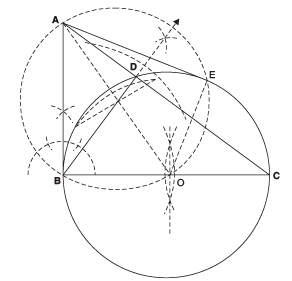Justification
Join OE, then ∠AEO = 90° [Angle being in a semi circle] ∴
AE ⊥ OE.
But OE is a radius of the given circle.
⇒ AE has to be a tangent to the circle.
Similarly, AB is also a tangent to the given circle.

Q 7. Draw a circle with the help of a bangle. Take a point outside the circle. Construct the pair of tangents from this point to the circle.
Sol. Steps of construction:
I. Draw the given circle using a bangle.
II. Take two non parallel chords PQ and RS of this circle.
III. Draw the perpendicular bisectors of PQ and RS such that they intersect at O.
Therefore, O is the centre of the given circle.
IV. Take a point P′ outside this circle.
V. Join OP′ and bisect it. Let M be the mid point of OP′.
VI. Taking M as centre and OM as radius, draw a circle. Let it intersect the given circle at A and B.
VII. Join P′A and P′B. Thus, P′A and P′B are the required two tangents.Justification:
Join OA and OB.
Since ∠OAP = 90°    [Angle in a semi-circle]
∴ PA ⊥ OA
Also OA is a radius.
∴ PA has to be a tangent to the given circle.
Similarly, PB is also a tangent to the given circle.

Offer running on EduRev: Apply code STAYHOME200 to get INR 200 off on our premium plan EduRev Infinity!

132 docs

,

,

,

,

,

,

,

,

,

,

,

,

,

,

,

,

,

,

,

,

,

;